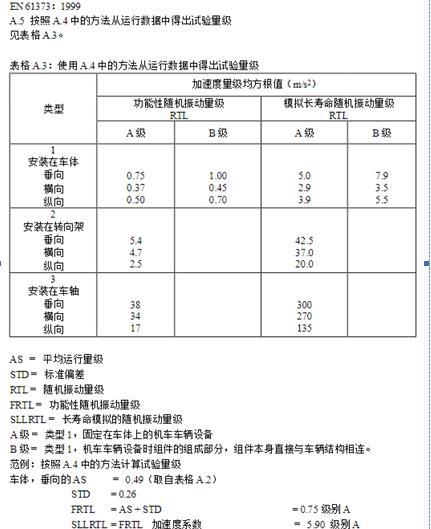# Blog&News

• Company News&Blog
• Catalog download
• Technical datasheet download

Company News&Blog

Your current location：Home - Blog&News - Company News&Blog

# EN61373:1999 Rail transit M12 x code D code connector

Time：2020-09-20 13:28:24　Hits： 291

EN61373:1999, where: is the service life; is the test time; is the running acceleration; is the test acceleration. By converting the above equation into the acceleration relationship, the following proportional formula is obtained: let the acceleration coefficient = time factor: where: 25% of the rated life; = 25 years of service life, 300 days / year, 10 hours / day, 25%; 18750 working hours, 5 hours of test time; equal to 4 (usually used for metals). The acceleration coefficient is 7.83, and the array data is collected for the application of this standard. The collected data are analyzed according to the root mean square magnitude, and the difference between the orders is marked as the standard deviation (see table A.3). Type 1, carbody, level B active random random vibration magnitude = average working magnitude + 2 standard deviation all other types of functional random vibration magnitude = average operation magnitude + 1 standard deviation long life simulation random vibration magnitude = functional random vibration magnitude acceleration coefficient (see test values calculated in table A.3)